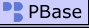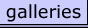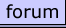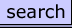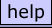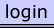A. J. deLange | all galleries >> Galleries >> miscellaneous > ResAlk.JPG
 previous | next

### ResAlk.JPG

Residual Alkalinity Chart: Residual Alkalinity is the portion of the alkalinity of a water sample that is not neutralized by the acid released when calcium reacts with malt phosphate. It is calculated as RA = [alk] - ([ca_hardness] + [mg_hardness]/2)/3.5. In this chart RA is set and the formula solved for the alkalinity which gives the set level of RA as a function of the hardness. Lines of constant RA are plotted and labeled with the corresponding expected mash pH under the assumption that a distilled water mash would produce a pH of 5.75. All units are ppm as CaCO3. Well known brewing cities are plotted on the chart. The lower left corner of the lable represents the water of the city. For example, Eeklo (Belgium) has water with alkalinity of about 205. Calcium plus half magnesium hardness is about 410.

To use the chart locate a point corresponding to the hardness and alkalinity of the water at hand. If the individual calcium and magnesium hardnesses are not known assume 60% of the total hardness is from calcium and 40% from magnesium (unless you know what the proportions tend to be for your area) and multiply the total hardness by 0.8. Read the RA from the nearest diagonal line. Interpolate visually between lines for a more refined answer (Eeklo's RA is about 90). If the plotted point is on or below the heavy line (0 RA) mash pH will probably be fine. If above the dotted line it will probably be too high. To estimate the amount of acid required to bring a given water to the heavy line measure the vertical (alaklinity) distance from the line. Divide the distance by 50 to get the number of milliequivalents per liter acid required to move to 0 RA. For example, Eeklo lies about 80 alkalinity units above the 0 RA line. Thus 1.6 mEq/L acid would be required to "neutralize" the residual alkalinity of this water. One could also increase the hardness of the water by 400 ppm as CaCO3 and reach the heavy line by moving horizontally. The fact that a couple of reported Burton sources are in this region idicates that water treated in this way would be similar to Burton water with repect to hardness and alkalinity. Or one could add 30/50 = 0.6 meE/L acid and increase hardness by 250 ppm to arive at the heavy line with water similar to Dortmund's.

other sizes: small medium original auto
 previous | next
 comment |